## Mozart's tuning: 55-EDO and its close relative, 1/6-comma meantone Text and diagrams © 2001 by Joseph L. Monzo with helpful comments by Paul Erlich

(You're listening to the beginning of my version of Mozart's 40th Symphony in G-minor, K. 550, tuned in a subset of 55-EDO.)

It is known that Leopold Mozart taught his son Wolfgang to use a meantone tuning where "flats" would be higher in pitch than "sharps". There is no documentation as to exactly what this tuning was, but there are a few clues.

Paul Erlich quoted in Yahoo Tuning List message 24125 (Thu May 31, 2001 9:03 pm) from Chesnut 1977 as follows:

Leopold Mozart refers to Tosi in general terms as an authoritative source in a letter to Wolfgang from Salzburg dated June 11, 1778. Tosi, in 1723, considered the correct tuning system to be what we would today call a form of regular meantone temperament ... according to Tosi, the large diatonic half step is theoretically equal to five ninths of a whole step, and the small chromatic half step is theoretically four-ninths of a whole step. Tosi thereby divides the octave into fifty-five equal parts. This is equivalent to tempering the perfect fifth by approximately one-sixth of a 'comma,' ...

Leopold Mozart, in his violin method of 1756 -- which happens to be the year of Wolfgang's birth -- also describes what we have called 'extended regular meantone temperament' as the correct intonation for the violin; he tells us that keyboard instruments of his time were played with some form of tempered [i.e., well-tempered] tuning, but that in the "right ratio" [i.e., meantone] tuning that he recommends for the violin, flats are higher by a comma than enharmonically equivalent sharps. It can be shown that for whichever of the standard commas we choose, the perfect fifths in Leopold Mozart's system were theoretically flattened by about one-sixth of that comma . . . Leopold Mozart wrote down a couple of scales specifically intended for practice in intonation, one leading through the flats, the other through the sharps. In practicing these scales, the student is supposed to learn to distinguish between the large diatonic half steps and the small chromatic half steps. It is important to emphasize that these scales are not abstractions but exercises to be mastered . . .

Thus it is apparent that for instruments other than keyboards (which both Mozart and his predecessors tuned in various well-temperaments, typically resembling 1/6-comma meantone for the "natural" notes, and Pythagorean for the "chromatic" keys), Mozart's tuning would be based on 55-EDO, or something very closely approximating it. It would have the following sizes for the basic intervals:

```
ratio    Semitones    cents

1 55-EDO degree               2(1/55)      12/55      21 9/11
smaller (chromatic) semitone  2(4/55)      48/55      87 3/11
larger (diatonic) semitone    2(5/55)     1 1/11     109 1/11
whole tone                    2(9/55)     1 53/55    196 4/11

```

55-EDO renders two different-sized semitones but only one size whole-tone; thus, it is a meantone. (Compare with the 50-EDO tuning recommended as a very close approximation to an "optimal meantone" by Woolhouse in 1835; and contrast with 53-EDO, which is so close to both Pythagorean and 5-limit JI that it gives two different-sized whole-tones.)

On this webpage I will first explore a 12-tone subset of 55-EDO, as I had mistakenly thought that it was the most likely intended tuning for Mozart's music. I have since learned that Mozart actually intended an interesting 20-tone subset of 55-EDO, and I give an update below on that. I decided to keep the info about the 12-tone subset because many people with ordinary Halberstadt keyboards may wish to explore that subset of 55-EDO, as it still gives a nice meantone system.

Note that for non-keyboard instruments, Mozart's actual conception of this tuning extended in a meantone cycle from Ebb to A#, with Cb omitted, for a total of 20 notes, thus giving two different pitches for the pairs of "sharp and "flat" notes (which are each one "black key" on the Halberstadt keyboard), and alternates for several of the "natural" notes too. So there is no evidence that Mozart ever actually used or advocated a 12-tone subset of this tuning (which is what I present here).

The mapping uses "D" as the symmetrical pitch center, and renders the 12-tone subset as a meantone "cycle of 5ths" from Eb to G#, with the "wolf" appearing between those two pitches.

Note that 55-EDO is audibly identical to 1/6-comma meantone. The "5th" in 55-EDO,

2(32/55) = ~698.181818... cents,
is equivalent to that of ~0.175445544-comma meantone. To describe that in terms of low-integer fraction-of-a-comma meantones, that's
• a little less than 1/5 (just slightly more than 3/16, extremely close to 7/37, and almost exactly 10/53) of a cent narrower than the 1/6-comma meantone "5th" = ~698.3706193 cents,

• even closer (less than 1/7 of a cent wider) to the 2/11-comma meantone "5th" = ~698.0447664 cents,

• much closer (~1/46-cent wider) to the 3/17-comma meantone "5th" = ~698.1597733 cents,

• closer still (less than 1/100-cent narrower) to the 7/40-comma meantone "5th" = ~698.1914002 cents,

• and almost bang on (~1/6692-cent wider) the 10/57-comma meantone "5th" = ~698.1819676 cents.
• The amount of tempering in 1/6-comma meantone is:

```
(81/80)(1/6) =  (2-4 * 34 * 5-1)(1/6) =  2(-2/3) * 3(2/3) * 5(-1/6)

= ~3.584381599 cents = ~3 & 3/5 cents.
```

So the 1/6-comma meantone "5th" is (3/2) / ((81/80)(1/6)). Using vector addition, that's:

```

2^    3^    5^

| -1     1     0   |    =  3/2
- | -2/3   2/3  -1/6 |    =  (81/80)(1/6)
-----------------------
| -1/3   1/3   1/6 |    =  1/6-comma meantone "5th"

= ~698.3706193 cents.

```

Using vector addition again to compare the 1/6-comma meantone "5th" with the 55-EDO "5th", we get:

```

2^     3^    5^

|   -1/3    1/3   1/6 |    =  1/6-comma meantone "5th"
- |   32/55   0     0   |    =  55-EDO "5th"
-------------------------
| -151/165  1/3   1/6 |    =  1/6-comma "5th" "-" 55-EDO "5th"

= ~0.188801084 cent = ~1/5 or ~10/53 cent, as stated above.

```

Below I present a side-by-side comparison of 55-EDO and 1/6-comma meantone.

First, the 12-out-of-55-EDO subset and the 12-tone 1/6-comma meantone presented as cycles of "5ths" from Eb to G#: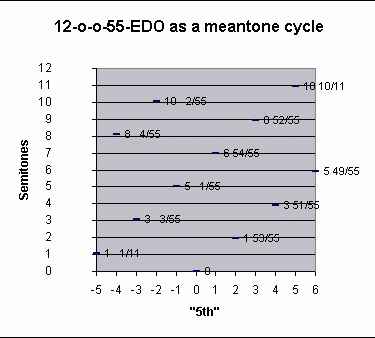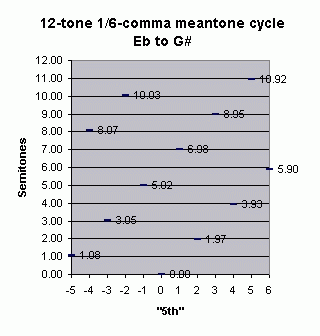Next, both of the scales presented as 12-tone chromatic scales, with their cycle-of-"5ths" and Semitone values, the Semitones and 55-EDO degrees between notes in the scale, and the 55-EDO degree representing the scale notes: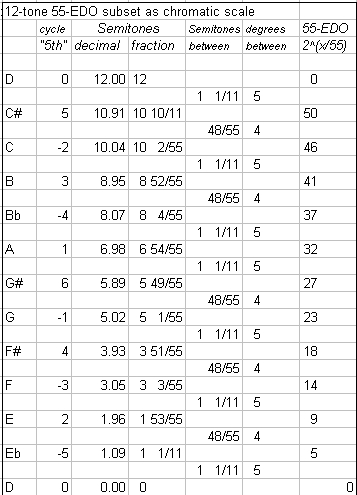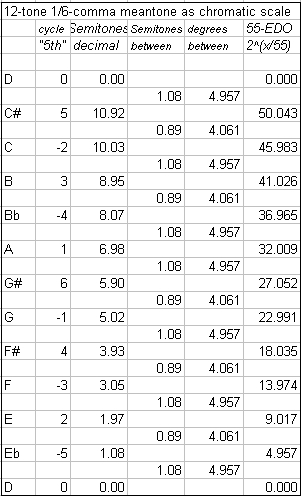And last, the interval matrices for both tunings, showing the 55-EDO degrees for the 12-o-o-55 subset and the "cycle-of-5ths" value for the 1/6-comma meantone, with the Semitone values of all possible intervals given in the body of the table. Some important intervals are color-coded for easy recognition: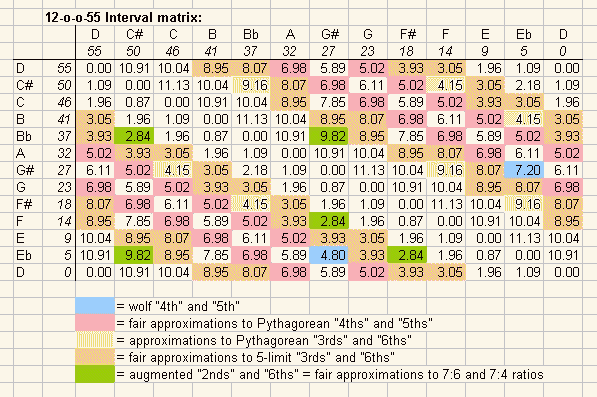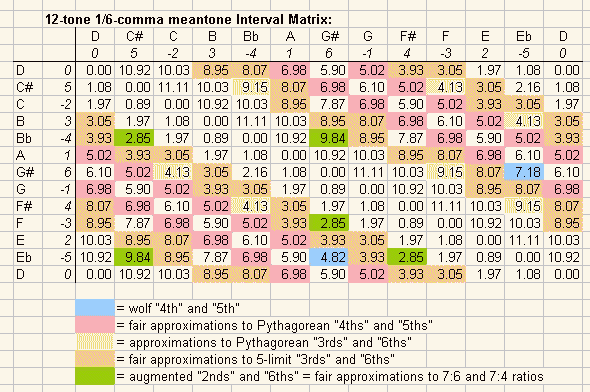Here is the above table reproduced with fractional cents values instead of decimal Semitones: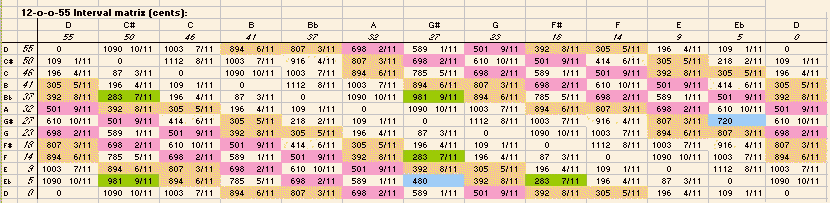Note also the following comment by Paul Erlich (from a Yahoo Tuning List post), echoing what Hind wrote at the end of his article:

There is no real evidence that Mozart intended 1/6- comma meantone rather than 1/5-comma or some other meantone system. It wouldn't make much sense for one to be that specific in regard to Mozart anyway, since he clearly assumes enharmonic equivalence even in a few passages for strings unaccompanied by keyboard, which violates his known violin teaching altogether.

## REFERENCE

Chesnut, John Hind. 1977.
"Mozart's teaching of intonation",
Journal of the American Musicological Society
vol. 30 no. 2 [summer], pp. 254-271.

section above updated: 2001.7.8, 2001.6.3

Here is a graph of a 56-tone cycle of 1/6-comma meantone, centered on "C" as the reference (= generator 0):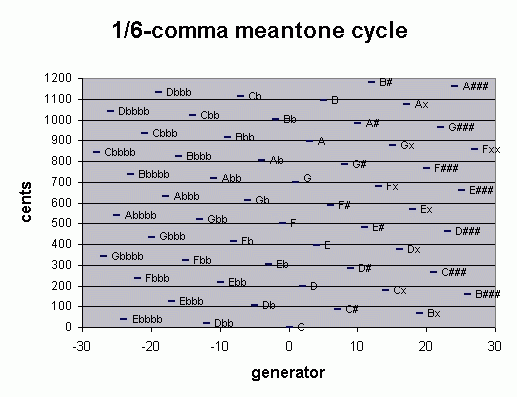As seen above, extending the cycle beyond 12 pitches results in pairs of pitches separated by approximately a comma. Upon passing beyond the -27..+27 cycle, note that the pitches represented as Cbbbb and Fxx are closer than that:

```

Fxx = ( (3/2)27 / ( (81/80)(27/5) ) ) / 215

=  2-(144/6) * 3(54/6) * 5(27/6)

=  ~856.0067202 cents

Cbbbb = ( (3/2)-28 / ( (81/80)(-28/5) ) ) / 212

=  2(158/6) * 3-(56/6) * 5-(28/6)

=  ~845.6226606 cents

2-(144/6) * 3(54/6) * 5(27/6)     Fxx
- 2(158/6) * 3-(56/6) * 5-(28/6)    Cbbbb
----------------------------
2-(302/6) * 3(110/6) * 5(55/6)    =  ~10.38405963 (= 10 ~3/8) cents.

10.38405963 / 55  =  0.188801084 --> compare with above.

```

Thus, limiting the meantone cycle to 55 pitches and distributing this difference equally among them, results in 55-EDO. If we call the 21 9/11 step-size of 55-EDO a "comma", then the "chromatic semitone" = 4 commas, the "diatonic semitone" = 5 commas, and the "whole-tone" = 9 commas, exactly as Mozart taught his students.

Other EDOs which approximate 1/6-comma meantone more closely than 55-EDO are 67- and 122-EDO:

```
2(39/67)  = ~698.5074627 cents
2(71/122) = ~698.3606557 cents
```

I'm adding a new section here which explores Mozart's actual intended tuning. Knowledge of this tuning is based on notes written down by Thomas Atwood, who studied with Mozart during the mid-1780s. My source for this is the Chesnut article cited above.

Mozart used the same process as given above, but extended it to a cycle of 21 meantone "5ths", an elegantly symmetrical system from implied 3-10 = Ebb to implied 310 = A#. He then destroyed the symmetry of the scale by limiting himself to 20 notes and eliminating the implied 3-7 = Cb.

It's curious to me that Mozart decided to do this, rather than keep the symmetrical tuning. It's interesting that the very note he leaves out is the one which would become the most characteristic feature of the later development (just after Mozart's death) of "expressive" Pythagorean-based intonation: the "raised" (sharpened or narrowed) "leading-tone" [again, see the Chesnut article for details].

Chesnut notes that Tosi advocated 55-EDO, and that one degrees or step in this tuning (i.e., the ratio 2(1/55) = 21 & 9/11 cents) is so close to both the syntonic and Pythagorean commas that it could be called a "Tosi comma". There are exactly 4 & 7/12 of these "Tosi commas" in one 12-EDO Semitone.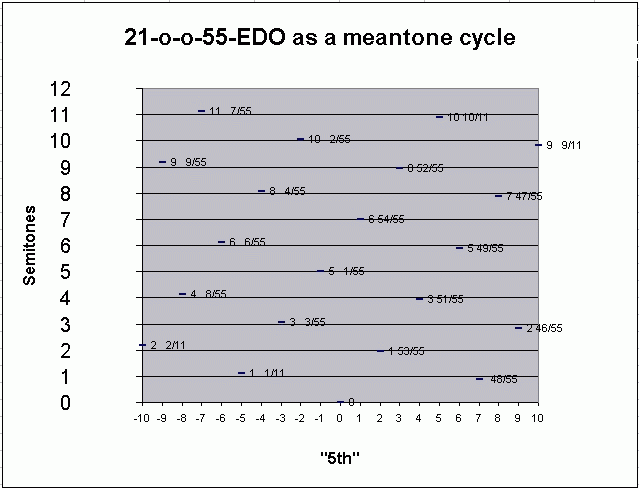Below is the mapping of the basic prime intervals in 55-EDO:

• "per" = number of instances of the period of equivalence needed for "correction" - in this case, "octaves" - to put the generated interval into the proper register to represent the basic prime interval.

• "gen" = number of 2(32/55) generators from 0. In this temperament, the generator is considered to be the "5th".

This notation thus gives the 55-EDO analogue of a vector notation using 2 (the "octave") and 3/2 (the "5th") as factors.

```

32/55-EDO mapping
prime   (per, gen)      ~cents error   ~ % error

2      ( 1, 0 )        0               0
3      ( 1, 1 )       -3.773182684    -0.198384435
5      ( 0, 4 )        6.413558862     0.230180788
7      (-3, 10)       12.99227535      0.385661821
11      (-7, 18)       15.95478491      0.384330594

```
• (For a detailed explanation of this type of matrix notation, see Graham Breed's matrix webpage. Graham would define 1/6-comma meantone as follows:

```
factors: 2    3    5                    implied ratio

( 1    0    0  )    (1200)          2:1
H' = ( 2/3  1/3  1/6)H = (1898) cents   ~3:1
(-4/3  4/3  2/3)    (2793)         ~5:1
```

And 55-EDO as follows:

```
factors:  2     3   5                    implied ratio

(  1     0   0)     (1200)          2:1
H' = ( 87/55  0   0)H  = (1898) cents   ~3:1
(128/55  0   0)     (2793)         ~5:1
```
)

Note that the generator which represents 11 lies outside the 21-tone cycle given in the above graph, thus, this particular scale only provides three approximate 11:8s, between the pairs of notes (expressed as generators and pitches where C = 0): (-10, 8) = Ebb:G#, (-9, 9) = Bbb:D#, and (-8, 10) = Fb:A#. This can be seen in the interval matrix further below.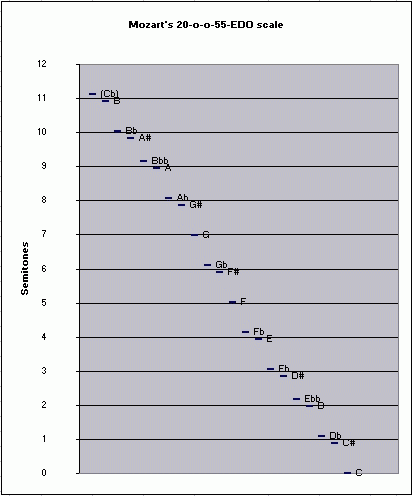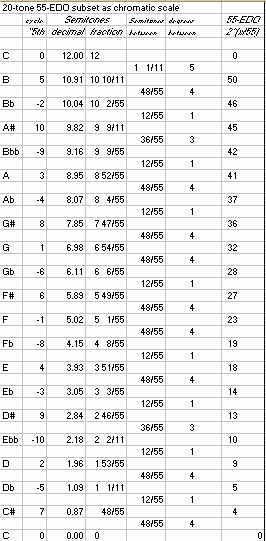Below is the interval matrix for Mozart's 20-out-of-55-EDO tuning, with intervals given in cents:Below is a lattice illustrating the relationship of 1/6-comma meantone with the 5-limit JI pitch-classes it implies. (This particular example illustrates a symmetrical 27-tone chain of 1/6-comma meantone "5th"s; it could be extended in either direction.)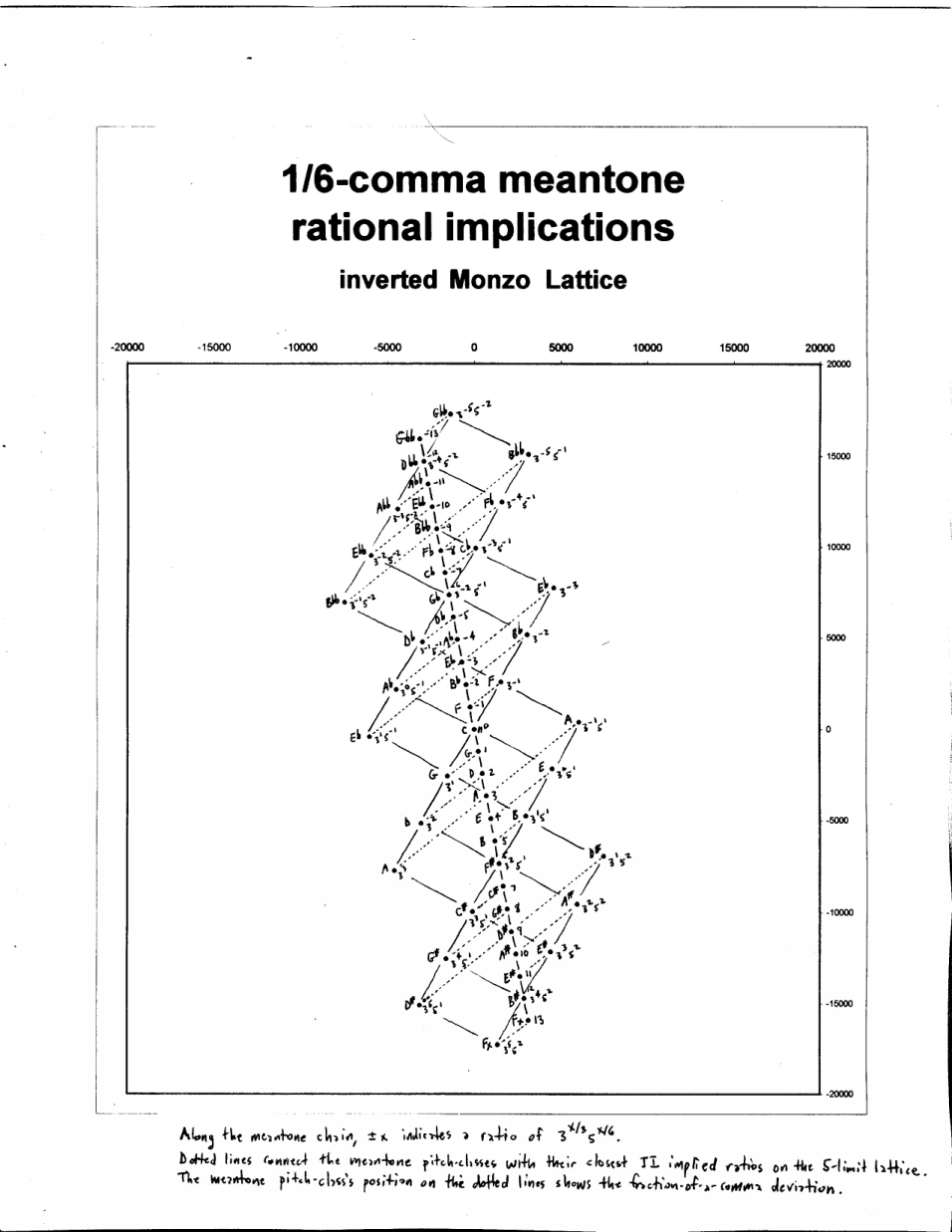Disclaimer: My intention in presenting the following two sections was to show how 55-EDO compares to 72-EDO and Pythagorean tuning. Upon further reflection, however, it turns out that neither of these two tunings approximates 55-EDO very well, since 55-EDO acts as a meantone and those two do not. Perhaps this material should be removed... but I'll leave it in for now; just take note of this disclaimer.

The table and graph below show the deviation of 72-EDO, which is gathering enthusiasm for adoption as a new tuning standard, from Mozart's 55-EDO subset. The table also shows the nearest 12-EDO pitch and the cawapus necessary to make CakewalkTM supply the proper tuning using pitch-bend in a MIDI-file.

Paul Erlich has criticized this comparison, since I originally claimed that 72-EDO provided a good approximation to Mozart's scale, and I did not take into consideration an examination of all the intervals. Note that in several cases there are two 72-EDO pitches which give roughly the same amount of error from 55-EDO, so I arbitrarily chose one where the other could have been chosen, and these choices will affect the interval matrix. In light of this, consider these graphs and tables to show the error for 72-EDO approximation of 55-EDO, as support for 72-EDO as a standard continues to grow.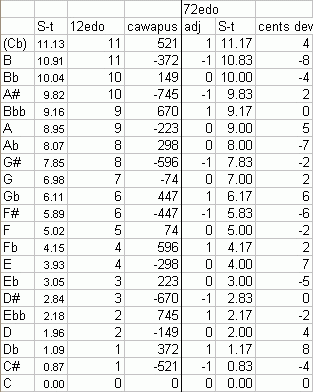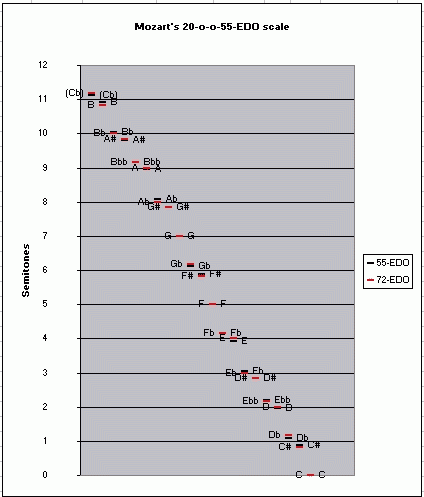Paul's criticism emphasized that one must consider not just the proximity of pitches in the two scales, but rather a comparison of all intervals occurring in the two tunings. So here is the interval matrix for this 20-tone subset of 72-EDO... compare it with the 20-tone subset of 55-EDO above. Paul doesn't consider 72-EDO to give a good approximation of any meantone or meantone-like tuning.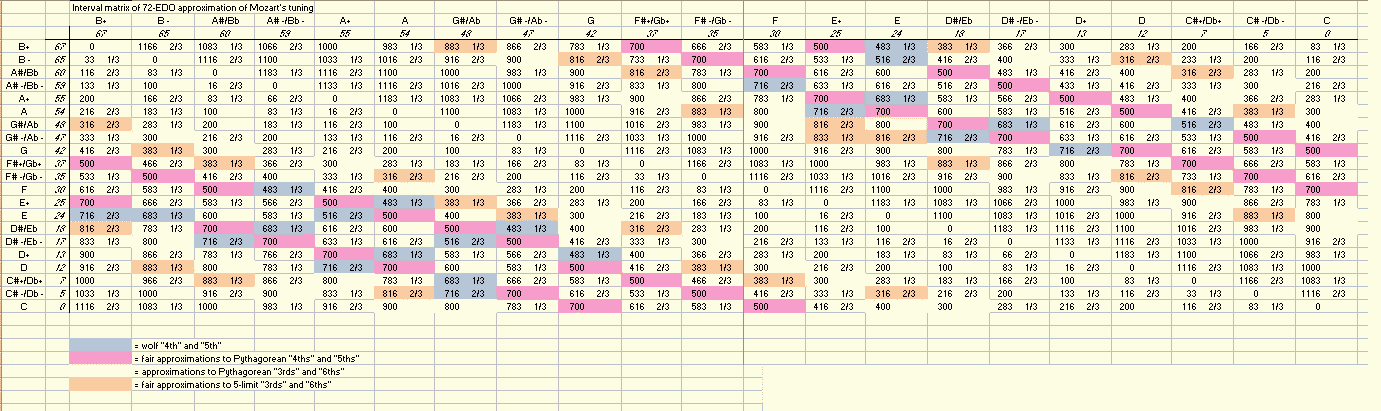. . . . . . . . . . . . . . . . . . . . . . . . . .

Mozart's 20-tone subset of 55-EDO can also be approximated by an extended Pythagorean system which is very similar to one proposed by Prosdocimus c. 1425, with the important difference that the meaning of the "flat" and "sharp" accidentals with respect to type of semitone is reversed. It is precisely because both meantone and Pythagorean tunings provide 2 different-sized semitones that they can spawn similar subsets like this one. But in the Pythagorean tuning the diatonic semitone (where the accidental sign doesn't change but the nominal does) is the smaller one, and the chromatic semitone (where the accidental sign changes but the nominal doesn't) is the larger one, whereas in the meantone tunings it is exactly the opposite.

Below is a table and graph showing the comparison between the 21-tone system from which Mozart's extracted his 20-tone subset of 55-EDO, and a 21-tone Pythgorean tuning which closely approximates it. Note that 19 tones of the Pythagorean system form a complete self-contained chain of "5ths", from 3-9 to 38, and that a big skip in the chain ocurrs at both ends, to include 3-14 and 314. I have more to say on this 19-tone subset below. Note that on the graph, if the blue plot-line for 55-EDO can't be seen, that's because the Pythgorean pitch approximates it so closely that it's covered.Paul Erlich also added in a private email to me:

19 is an MOS of the meantone-fifth generator. Hence it will be a CS -- any given specific interval will always span the same number of degrees.

Paul suggested that I make an interval matrix of the 19-tone subset, which I would spell with D again at the center for symmetry, and which would thus extend from 3-9 = Cb to 39 = E#... hopefully I'll get around to it. It was more important for me to explore the historical ramifications of Mozart's actual tuning.

last section updated: 2001.6.5, 2001.6.28

 For many more diagrams and explanations of historical tunings, see my book. If you don't understand my theory or the terms I've used, start here or try some definitions.

 I welcome feedback about this webpage: corrections, improvements, good links. Let me know if you don't understand something.return to my home pagereturn to the Sonic Arts home page